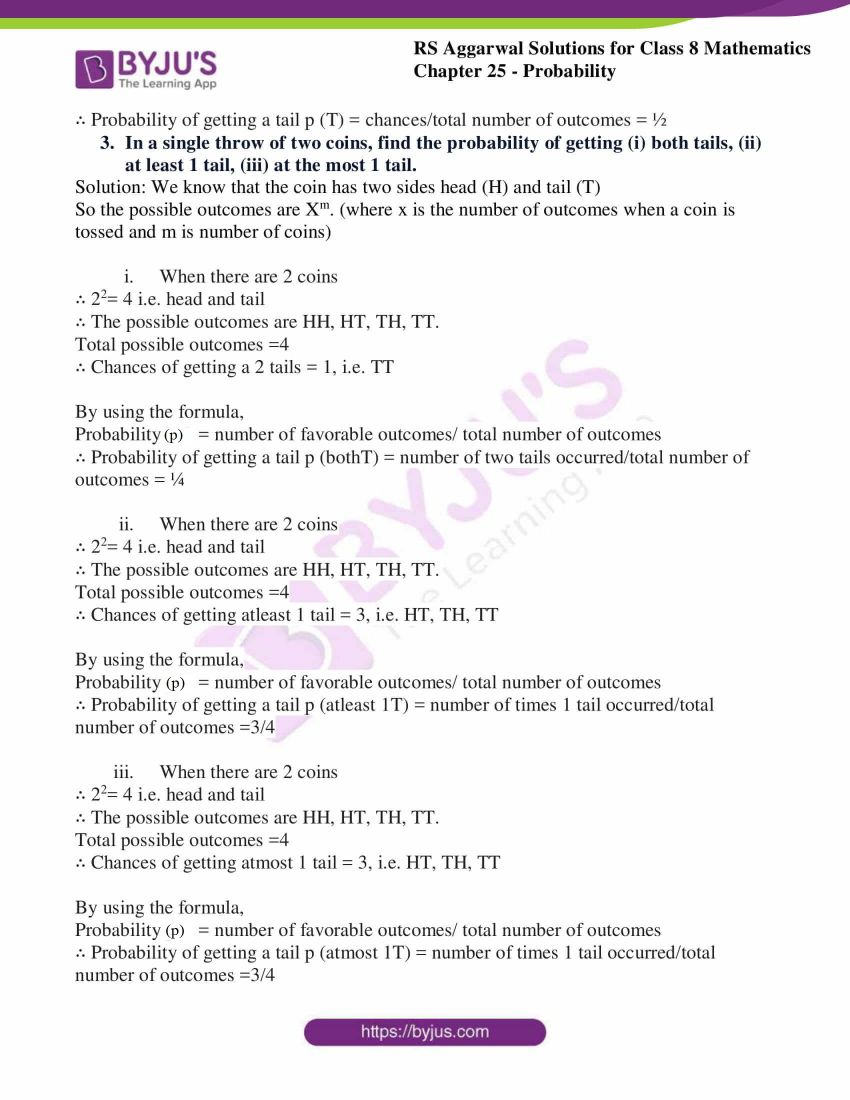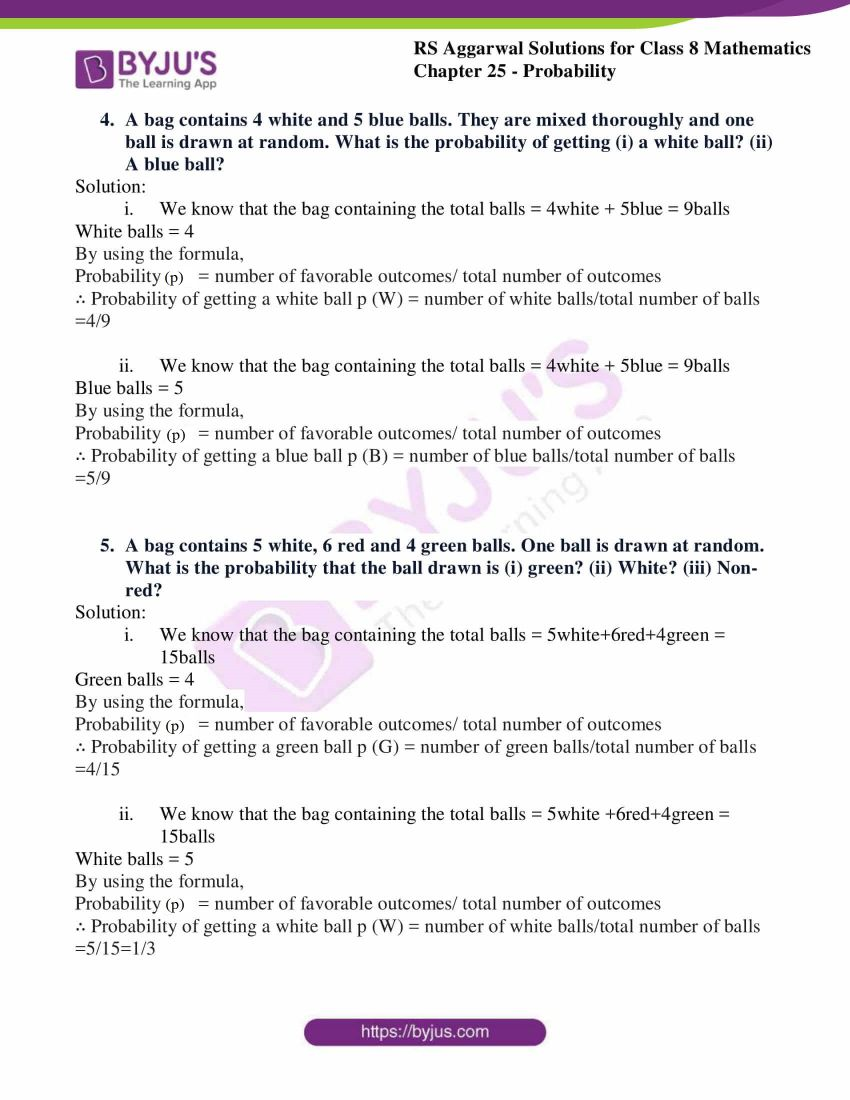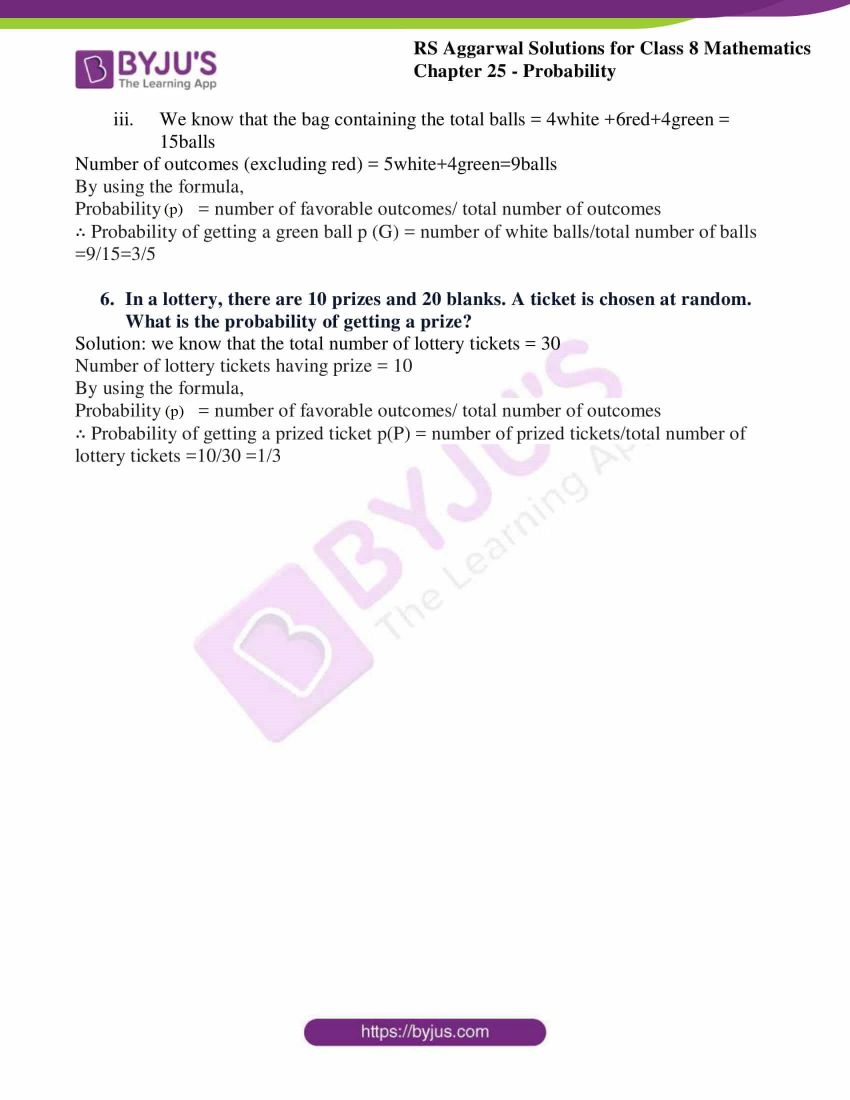# RS Aggarwal Solutions for Class 8 Maths Chapter 25 - Probability Exercise 25A

Download RS Aggarwal Solutions for Class 8 Maths Chapter 25- Exercise 25A, Probability from the links provided below. Our expert tutors have solved the RS Aggarwal Solutions in an easily understandable manner which helps students secure high marks. In the solutions of the questions present in Exercise 25A, of RS Aggarwal Class 8 Maths, the probability of occurrence of an event with some experiments and their outcomes are discussed.

## Download PDF of RS Aggarwal Solutions for Class 8 Maths Chapter 25- Probability Exercise 25A### Access Answers to RS Aggarwal Solutions for Class 8 Maths Chapter 25- Probability Exercise 25A

Q1.
(i) A coin is tossed. What are all possible outcomes?
(ii) Two coins are tossed simultaneously. What are all possible outcomes?
(iii) A die is thrown. What are all possible outcomes?
(iv) From a well-shuffled deck of 52 cards, one card is drawn at random. What is the number of all possible outcomes?
Solution: We know that the coin has two sides head (H) and tail (T)
So the possible outcomes are Xm. (where x is the number of outcomes when a coin is tossed and m is number of coins)
(i) ∴ 21= 2 i.e. head and tail
∴ The possible outcomes are H and T.

(ii) When there are 2 coins
∴ 22= 4 i.e. head and tail
∴ The possible outcomes are HH, HT, TH, TT.

(iii) We know that the die has 6 facesSo, they are 1, 2, and 3,4,5,6
∴ The possible outcomes are 1, 2, 3,4,5,6.

(iv) We know that the deck of cards has a total of 52 cardsSo, they are 52cards
∴ The possible outcomes are 52cards.

2. In a single throw of coin, what is the probability of getting a tail?
Solution:
We know that the coin has two sides head (H) and tail (T)
So the possible outcomes are Xm.
(where x is the number of outcomes when a coin is tossed and m is number of coins)
∴ The possible outcomes are H and T.
Total possible outcomes =2
∴ Chances of getting a tail = 1(since there is a single coin)
By using the formula,
Probability (p) = number of favorable outcomes/ total number of outcomes
∴ Probability of getting a tail p (T) = chances/total number of outcomes = ½

3. In a single throw of two coins, find the probability of getting (i) both tails, (ii) at least 1 tail, (iii) at the most 1 tail.
Solution: We know that the coin has two sides head (H) and tail (T)
So the possible outcomes are Xm.
(where x is the number of outcomes when a coin is tossed and m is number of coins)

(i) When there are 2 coins∴ 22= 4 i.e. head and tail
∴ The possible outcomes are HH, HT, TH, TT.
Total possible outcomes =4
∴ Chances of getting a 2 tails = 1, i.e. TT
By using the formula,
Probability (p) = number of favorable outcomes/ total number of outcomes
∴ Probability of getting a tail p (bothT) = number of two tails occurred/total number of outcomes = ¼

(ii) When there are 2 coins∴ 22= 4 i.e. head and tail
∴ The possible outcomes are HH, HT, TH, TT.
Total possible outcomes =4
∴ Chances of getting atleast 1 tail = 3, i.e. HT, TH, TT
By using the formula,
Probability (p) = number of favorable outcomes/ total number of outcomes
∴ Probability of getting a tail p (atleast 1T) = number of times 1 tail occurred/total number of outcomes =3/4

(iii) When there are 2 coins∴ 22= 4 i.e. head and tail
∴ The possible outcomes are HH, HT, TH, TT.
Total possible outcomes =4
∴ Chances of getting atmost 1 tail = 3, i.e. HT, TH, TT
By using the formula,
Probability (p) = number of favorable outcomes/ total number of outcomes
∴ Probability of getting a tail p (atmost 1T) = number of times 1 tail occurred/total number of outcomes =3/4

4. A bag contains 4 white and 5 blue balls. They are mixed thoroughly and one ball is drawn at random. What is the probability of getting (i) a white ball? (ii) A blue ball?
Solution:

(i) We know that the bag containing the total balls ,
= 4white + 5blue = 9ballsWhite balls = 4
By using the formula,
Probability (p) = number of favorable outcomes/ total number of outcomes
∴ Probability of getting a white ball p (W) = number of white balls/total number of balls =4/9

(ii) We know that the bag containing the total balls = 4white + 5blue = 9ballsBlue balls = 5
By using the formula,
Probability (p) = number of favorable outcomes/ total number of outcomes
∴ Probability of getting a blue ball p (B) = number of blue balls/total number of balls =5/9

5. A bag contains 5 white, 6 red and 4 green balls. One ball is drawn at random. What is the probability that the ball drawn is (i) green? (ii) White? (iii) Non-red?
Solution:

(i) We know that the bag containing the total balls = 5white+6red+4green = 15ballsGreen balls = 4
By using the formula,
Probability (p) = number of favorable outcomes/ total number of outcomes
∴ Probability of getting a green ball p (G) = number of green balls/total number of balls =4/15

(ii) We know that the bag containing the total balls = 5white +6red+4green = 15ballsWhite balls = 5
By using the formula,
Probability (p) = number of favorable outcomes/ total number of outcomes
∴ Probability of getting a white ball p (W) = number of white balls/total number of balls =5/15=1/3

(iii) We know that the bag containing the total balls = 4white + 6red + 4green = 15ballsNumber of outcomes (excluding red) = 5white+4green=9balls
By using the formula,
Probability (p) = number of favorable outcomes/ total number of outcomes
∴ Probability of getting a green ball p (G) = number of white balls/total number of balls =9/15=3/5

6. In a lottery, there are 10 prizes and 20 blanks. A ticket is chosen at random. What is the probability of getting a prize?
Solution: we know that the total number of lottery tickets = 30
Number of lottery tickets having prize = 10
By using the formula,
Probability (p) = number of favorable outcomes/ total number of outcomes
∴ Probability of getting a prized ticket p(P) = number of prized tickets/total number of lottery tickets =10/30 =1/3

### Access other Exercise of RS Aggarwal Solutions for Class 8 Maths Chapter 25- Probability

Exercise 25B Solutions 8 Questions

## RS Aggarwal Solutions for Class 8 Maths Chapter 25- Probability Exercise 25A

Exercise 25A of RS Aggarwal Class 8, Probability, these exercises mainly consists of questions related to the Probability which includes some experiments like tossing a coin, tossing two coins, and throwing a die results in their respective outcomes. In the RS Aggarwal Solutions book, many such exercise problems are given, which enhance familiarity with the concepts. Students are suggested to practice the problems regularly, which will help them excel in their exams and increase their overall percentage. Practising as many times as possible helps in building time management skills and also boosts the confidence level to achieve high marks.